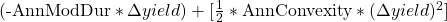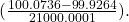Understanding Fixed-Income Risk and Return | IFT World
IFT Notes for Level I CFA® Program
IFT Notes for Level I CFA® Program

# Part 5

## 10. Investment Horizon, Macaulay Duration and Interest Rate Risk

In this section, we look at how yield volatility affects the investment horizon. We also study the interaction between the investment horizon, market price risk, and coupon reinvestment risk.

### 10.1 Yield Volatility

If there is a change in a bond’s YTM, there will be a corresponding change in the price of a bond. The change in the price can be explained as the product of two factors:

1. Price value of a basis point (PVBP): Impact on bond price of a one basis point change in YTM. This factor is based on the duration and convexity of the bond.
2. Number of basis points: This is the change in yield measured in basis points.

The percentage change in the price of a bond for a given change in yield can also be determined using this equation:

%ΔPVFULL =Example 17: Ranking bonds in terms of interest rate risk

A fixed-income analyst is asked to rank three bonds in terms of interest rate risk. The increases in the yields-to-maturity represent the “worst case” for the scenario being considered.

 Bond Modified Duration Convexity ∆Yield A 3.65 14.8 50 bps B 5.75 38.7 25 bps C 12.28 146 15 bps

The modified duration and convexity statistics are annualized. ∆Yield is the increase in the annual yield-to-maturity. Rank the bonds in terms of interest rate risk.

Solution:

Calculate the estimated price change for each bond:

Bond A:

The duration effect is -3.65 × 0.005 = -1.825%.

The convexity effect is 0.5 × 14.8 × 0.005^2= 0.0185%.

The expected change in bond price is -1.825% + 0.0185% = -1.8065%.

Bond B:

The duration effect is -5.75 × 0.0025 = -1.4375%.

The convexity effect is 0.5 × 38.7 × 0.0025^2= 0.0121%.

The expected change in bond price is -1.4375% + 0.0121% = -1.4254%.

Bond C:

The duration effect is -12.28 × 0.0015 = -1.842%.

The convexity effect is 0.5 × 146 × 0.0015^2= 0.0164%.

The expected change in bond price is -1.842% + 0.0164% = -1.8256%.

Bond C has the highest degree of interest rate risk (a potential loss of 1.8256%), followed by Bond A (a potential loss of 1.8065%) and Bond B (a potential loss of 1.4254%).

### 10.2 Investment Horizon, Macaulay Duration, and Interest Rate Risk

The impact of a sudden change in yield on the price of a bond is of particular concern to short-term investors (price risk). Long term investors will also be concerned about the impact of a change in yield on the reinvestment income (reinvestment risk). An investor who plans to hold the bond to maturity will only be concerned about reinvestment risk.

Consider another 10-year, 8% annual coupon bond priced at 85.5 and YTM of 10.4%. If the investment horizon is 10 years, the only concern is reinvestment risk. Consider a scenario where interest rates go down. In this case, reinvestment income goes down. When the price of the bond goes up, it does not matter to the investor because at maturity he will simply receive par value.

When interest rates go up, the reinvestment income goes up. If investment horizon is 4 years, then the major concern is price risk. In this case, the price effect dominates relative to the gain/loss from reinvestment of coupons. If the investment horizon is 7 years, the reinvestment risk and price risk offset each other.  For this particular bond the Macaulay duration is 7 years.

Macaulay duration indicates the investment horizon for which coupon reinvestment risk and market price risk offset each other. The assumption is a one-time parallel shift in the yield curve.

Note: Investment horizon is different from the bond’s maturity. In this case, the maturity is 10 years while the horizon is 7 years.

The duration gap of a bond a bond is defined as the Macaulay duration – investment horizon.

Duration gap = Macaulay duration – Investment horizon

• If Macaulay duration < investment horizon, the duration gap is negative: coupon reinvestment risk dominates.
• If investment horizon = Macaulay duration, the duration gap is zero: coupon reinvestment risk offsets market price risk.
• If Macaulay duration > investment horizon, the duration gap is positive: market price risk dominates.

Example 18: Calculating duration gap and assessing interest rate risk

An investor plans to retire in 8 years. As part of the retirement portfolio, the investor buys a newly issued, 10-year, 6% annual coupon payment bond. The bond is purchased at par value, so its yield-to-maturity is 6.00% stated as an effective annual rate.

1. Calculate the approximate Macaulay duration for the bond, using a 1 bp increase and decrease in the yield-to-maturity and calculating the new prices per 100 of par value.
2. Calculate the duration gap at the time of purchase.
3. Does this bond at purchase entail the risk of higher or lower interest rates? Interest rate risk here means an immediate, one-time, parallel yield curve shift.

Solution to 1:

The approximate modified duration of the bond is 7.36 =PV0= 100,  PV+= 99.9264

FV = 100, PMT = 6, I/Y = 6.01, N = 10, CPT PV, PV = -99.9264

PV_ = 100.0736; FV = 100, PMT = 6, I/Y = 5.99, N = 10, CPT PV, PV = -100.0736.

The approximate Macaulay duration = 7.36 × 1.06 = 7.8016.

Solution to 2:

Given an investment horizon of 8 years, the duration gap for this bond at purchase is negative: 7.8016 – 8 = -0.1984

Solution to 3:

A negative duration gap entails the risk of lower interest rates. To be precise, the risk is an immediate, one-time, parallel, downward yield curve shift because the coupon reinvestment risk dominates market price risk. The loss from reinvesting coupons at a rate lower than 6% is larger than the gain from selling the bond at a price above the constant-yield price trajectory.

LIVE WEEKEND CLASSES *For 2024 Exam candidates*
This is default text for notification bar